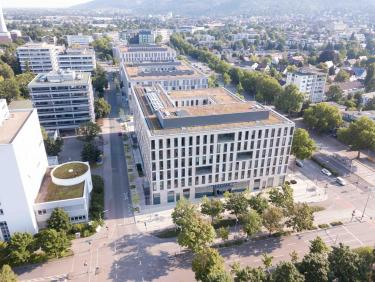# SectionAnalysis and Mathematical Modelling

Partial differential equations and ordinary differential equations are the foundational language in which many systems from physics, biology, engineering and many other sciences are modelled. The examples include reaction-diffusion systems of pattern formation, continuum mechanics models of elasticity and fluid dynamics, models for electro- and magnetostatics and structured population models.

In Heidelberg we are interested in the modelling and analysis of complex systems involving multiple scales and parameters that control the emergence of nonlocal and nonlinear effects. In particular, we explore a range of pattern formation mechanisms arising from nonconvexity or hysteresis. To tackle these problems, we use tools and ideas from different areas such as asymptotic and singular perturbation analysis, semigroup theory, energy methods, harmonic and microlocal analysis. These mathematical techniques are also applied to derive effective model equations and to study their dynamics in dependence of model variables and parameters.## Key Questions

Key questions in the understanding of these systems are:

1. Is the problem well-posed in the sense that it admits a solution in a desirable functional space? Is the solution unique?
2. What do we know about the properties of the solution such as its dependence on initial conditions and model parameters?
3. Can we understand the effective behaviour of the system in the terms of its parameters?

## Our research interests

In the research group of H. Knüpfer, we investigate models from materials science and fluid dynamics. In particular, we are interested in the analysis of pattern formation in non-convex systems with nonlocal interaction such as ferromagnetic systems, systems with long-range Riesz type interaction or elastic materials. Another interest are free boundary problems in fluid dynamics. Information about the group members and details reseach and teaching can be found here.

The interests of the research group of A. Rüland revolve around inverse problems, nonlocal elliptic equations, free boundary value problems and the calculus of variations. It is the group's aim to analyse important applications from the sciences with mathematically innovative tools. Information on the group's current structure, research interests and teaching portfolio can be found here.

The interdisciplinary expertise of the research group of A. Marciniak-Czochra lies in the areas of applied mathematics and mathematical biosciences. Our field of focus is the dynamics of self-organisation and structure formation in developmental and regeneration processes, and in cancer. The models developed in a close collaboration with experimentalists, are applied to investigate evolution and impacts of cellular heterogeneity in stem cell-based systems, systemic organisation of cell fate control and mechanisms of symmetry breaking and pattern formation. The mathematical areas of focus are partial differential equations, dynamical systems and multiscale analysis. The main lines of analytical research are devoted to (1) emergence and stability of pattern formation in reaction-diffusion type equations;  (2) analysis of nonlinear structured population models; linking continuous and discrete structures; (3) derivation of effective models starting from first-principle modelling to describe growth and transport processes. Particular attention is paid to methods of model upscaling and reduction.